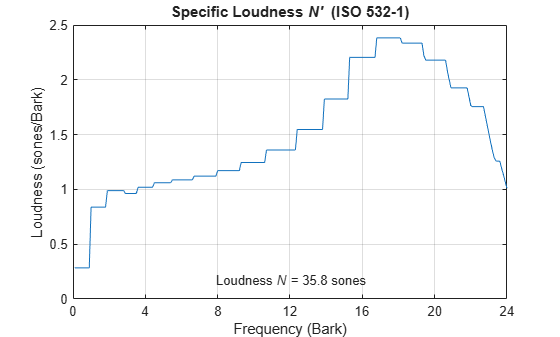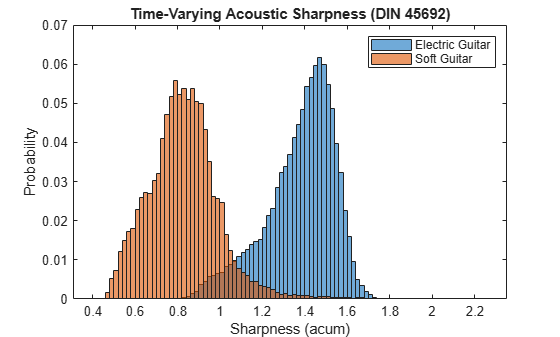# acousticSharpness

Perceived sharpness of acoustic signal

## Syntax

``sharpness = acousticSharpness(audioIn,fs)``
``sharpness = acousticSharpness(audioIn,fs,calibrationFactor)``
``sharpness = acousticSharpness(SPLIn)``
``sharpness = acousticSharpness(specificLoudnessIn)``
``sharpness = acousticSharpness(___,Name,Value)``
``acousticSharpness(___,TimeVarying,true)``

## Description

example

````sharpness = acousticSharpness(audioIn,fs)` returns sharpness in acum according to DIN 45692  and ISO 532-1:2017(E) .```
````sharpness = acousticSharpness(audioIn,fs,calibrationFactor)` specifies a nondefault microphone calibration factor used to compute loudness.```

example

````sharpness = acousticSharpness(SPLIn)` computes sharpness using one-third-octave-band sound pressure levels (SPL).```

example

````sharpness = acousticSharpness(specificLoudnessIn)` computes sharpness using specific loudness.```

example

````sharpness = acousticSharpness(___,Name,Value)` specifies options using one or more `Name,Value` pair arguments.Example: ```sharpness = acousticSharpness(audioIn,fs,calibrationFactor,'SoundField','diffuse')``` returns sharpness assuming a diffuse sound field.```

example

````acousticSharpness(___,TimeVarying,true)` with no output arguments plots sharpness relative to time.```

## Examples

collapse all

Compute the acoustic sharpness of turbine noise. Assume it is stationary and was recorded in a diffuse sound field.

```[audioIn,fs] = audioread('Turbine-16-44p1-mono-22secs.wav'); sharpness = acousticSharpness(audioIn,fs,'SoundField','diffuse'); fprintf('Acoustic sharpness = %0.2f acum\n',sharpness)```
```Acoustic sharpness = 1.11 acum ```

`[audioIn,fs] = audioread('RockDrums-48-stereo-11secs.mp3');`

Plot the time-varying sharpness of the signal. Listen to the signal.

`acousticSharpness(audioIn,fs,'TimeVarying',true)``sound(audioIn,fs)`

Create two stationary signals with equivalent power: a pink noise signal and a white noise signal.

```fs = 48e3; dur = 5; pnoise = 2*pinknoise(dur*fs); wnoise = rand(dur*fs,1) - 0.5; wnoise = wnoise*sqrt(var(pnoise)/var(wnoise));```

Call `acousticLoudness` using the default ISO 532-1 (Zwicker) method and no output arguments to plot the loudness of the pink noise. Call `acousticLoudness` again, this time with output arguments, to get the specific loudness.

```figure acousticLoudness(pnoise,fs)````[~,pSpecificLoudness] = acousticLoudness(pnoise,fs);`

Plot the loudness for the white noise signal and then get the specific loudness values.

```figure acousticLoudness(wnoise,fs)````[~,wSpecificLoudness] = acousticLoudness(wnoise,fs);`

Call the `acousticSharpness` function to compare the sharpness of the pink noise and white noise.

```pSharpness = acousticSharpness(pSpecificLoudness); wSharpness = acousticSharpness(wSpecificLoudness); fprintf('Sharpness of pink noise = %0.2f acum\n',pSharpness)```
```Sharpness of pink noise = 2.00 acum ```
`fprintf('Sharpness of white noise = %0.2f acum\n',wSharpness)`
```Sharpness of white noise = 2.62 acum ```

Create a pink noise signal with a 48 kHz sample rate and a duration of 5 seconds.

```fs = 48e3; n = fs*5; pnoise = pinknoise(n);```

Specify a vector to sweep over the dB range from -60 to 20. Create a gain vector which, when multiplied by the original signal, results in a signal with the desired output level.

```dBSweep = -60:10:20; coefSweep = sqrt((10.^(dBSweep/10))/var(pnoise));```

Call `acousticSharpness` in a loop with the different signal levels. Determine the sharpness using the default DIN 45692 frequency weighting and the Aures frequency weighting.

```sharpnessDIN45692 = zeros(numel(dBSweep),1); sharpnessAures = zeros(numel(dBSweep),1); for ii = 1:numel(dBSweep) signal = pnoise*coefSweep(ii); sharpnessDIN45692(ii) = acousticSharpness(signal,fs); sharpnessAures(ii) = acousticSharpness(signal,fs,'Weighting','Aures'); end```

Display the effect of the input level on the acoustic sharpness. The Aures frequency weighting method is more sensitive to the input level.

```plot(dBSweep,sharpnessDIN45692,dBSweep,sharpnessAures) legend('Weighting = DIN45692','Weighting = Aures') xlabel('Input Level (dB)') ylabel('Sharpness (acum)') title('Effect of Input Level on Sharpness') axis([dBSweep(1) dBSweep(end) 0 20]) grid on```Read in two audio files: one of an electric guitar with distortion and one of an acoustic guitar. Both audio files have a sample rate of 44.1 kHz. For easy comparison, convert the rock guitar signal to mono and shorten the soft guitar signal to the length of the rock guitar signal.

```fs = 44.1e3; rockGuitar = audioread('RockGuitar-16-44p1-stereo-72secs.wav'); softGuitar = audioread('SoftGuitar-44p1_mono-10mins.ogg'); rockGuitar = mean(rockGuitar,2); softGuitar = softGuitar(1:numel(rockGuitar));```

Calculate the time-varying sharpness for both the rock guitar and soft guitar.

```rGSharpness = acousticSharpness(rockGuitar,fs,'TimeVarying',true); sGSharpness = acousticSharpness(softGuitar,fs,'TimeVarying',true);```

Plot the probability distribution based on the observed sharpness of the rock guitar and the soft guitar.

```histogram(rGSharpness,'Normalization','probability') hold on histogram(sGSharpness,'Normalization','probability') legend('Electric Guitar','Soft Guitar') xlabel('Sharpness (acum)') ylabel('Probability') title('Time-Varying Acoustic Sharpness (DIN 45692)')````[audioIn,fs] = audioread('Turbine-16-44p1-mono-22secs.wav');`

To calculate sound pressure levels from an audio signal, first create an `splMeter` object. Call the `splMeter` object with the audio input.

```spl = splMeter("SampleRate",fs,"Bandwidth","1/3 octave", ... "FrequencyWeighting","Z-weighting","OctaveFilterOrder",6); splMeasurement = spl(audioIn);```

Compute the mean SPL level, skipping the first 0.2 seconds. Only keep the bands from 25 Hz to 12.5 kHz (the first 28 bands).

`SPLIn = mean(splMeasurement(ceil(0.2*fs):end,1:28));`

To determine the acoustic sharpness of the audio signal, call `acousticSharpness` using the sound pressure level input.

`sharpness = acousticSharpness(SPLIn)`
```sharpness = 1.1020 ```

## Input Arguments

collapse all

Audio input, specified as a column vector (mono) or matrix with two columns (stereo). Sharpness is computed for each channel (column) independently.

Data Types: `single` | `double`

Sample rate in Hz, specified as a positive scalar. The recommended sample rate for new recordings is 48 kHz.

Note

The minimum acceptable sample rate is 8 kHz.

Data Types: `single` | `double`

Microphone calibration factor, specified as a positive scalar. The default calibration factor corresponds to a full-scale 1 kHz sine wave with a sound pressure level of 100 dB (SPL). To compute the calibration factor specific to your system, use the `calibrateMicrophone` function.

Data Types: `single` | `double`

Sound pressure level (SPL) in dB, specified as a 1-by-28-by-C array. 28 corresponds to one-third-octave bands between 25 Hz and 12.5 kHz. C is the number of channels.

Data Types: `single` | `double`

Specific loudness in sones/Bark, specified as a T-by-240-by-C array, where:

• T is 1 for stationary signals or one per 2 ms for time-varying signals.

• 240 is the number of Bark bins in the domain for specific loudness. The Bark bins are `0.1:0.1:24`.

• C is the number of channels.

You can use the `acousticLoudness` function to calculate `specificLoudnessIn` using this syntax:

`[~,specificLoudnessIn] = acousticLoudness(audioIn,fs);`

Data Types: `single` | `double`

### Name-Value Pair Arguments

Specify optional comma-separated pairs of `Name,Value` arguments. `Name` is the argument name and `Value` is the corresponding value. `Name` must appear inside quotes. You can specify several name and value pair arguments in any order as `Name1,Value1,...,NameN,ValueN`.

Example: ```acousticSharpness(audioIn,fs,'Weighting','von Bismarck')```

Frequency weighting, specified as `'DIN 45692'`, `'Aures'`, or `'von Bismarck'`. By design, the `'Aures'` frequency weighting method is more sensitive to amplitude levels and proper calibration. For details, see Algorithms.

Data Types: `char` | `string`

Sound field of audio recording, specified as `'free'` or `'diffuse'`.

Data Types: `char` | `string`

Reference pressure for dB calculation in pascals, specified as a positive scalar. The default value, 20 micropascals, is the common value for air.

Data Types: `single` | `double`

Input is time-varying, specified as `true` or `false`. If `TimeVarying` is set to `true`, acoustic sharpness is calculated in 2 ms intervals.

Data Types: `logical`

## Output Arguments

collapse all

Acoustic sharpness in acum, returned as a scalar, vector, or matrix. Sharpness is computed according to DIN 45692 and ISO 532-1.

Data Types: `single` | `double`

## Algorithms

Acoustic sharpness is a measurement derived from acoustic loudness. The acoustic loudness algorithm is described in  and implemented in the `acousticLoudness` function. The acoustic sharpness calculation is described in . The algorithm for acoustic sharpness is outlined as follows.

`$sharpness\text{\hspace{0.17em}}=\text{\hspace{0.17em}}k\text{\hspace{0.17em}}\left(\frac{\underset{z=0}{\overset{24}{\int }}N\text{'}\left(z\right)\text{\hspace{0.17em}}g\left(z\right)\text{\hspace{0.17em}}z\text{\hspace{0.17em}}\text{d}z}{\underset{z=0}{\overset{24}{\int }}N\text{'}\left(z\right)\text{\hspace{0.17em}}\text{d}z}\right)$`

Where N' is the specific loudness in sones/Bark. The function g(z) and the scaling factor k depend on the specified `Weighting` method:

`'DIN 45692'`: k is set such that a 1 kHz reference tone results in a 1 acum sharpness measurement, and

`$\begin{array}{lll}g\left(z\right)=1\hfill & \text{for}\hfill & z\le 15.8\text{\hspace{0.17em}}\text{Bark}\hfill \\ g\left(z\right)=0.15{e}^{0.42\left(z-15.8\right)}+0.85\hfill & \text{for}\hfill & z>15.8\text{​}\text{\hspace{0.17em}}\text{Bark}\hfill \end{array}$`

`'von Bismark'`: k is set to `0.11`, and

`$\begin{array}{lll}g\left(z\right)=1\hfill & \text{for}\hfill & z\le 15\text{\hspace{0.17em}}\text{Bark}\hfill \\ g\left(z\right)=0.2{e}^{0.308\left(z-15\right)}+0.8\hfill & \text{for}\hfill & z>15\text{​}\text{\hspace{0.17em}}\text{Bark}\hfill \end{array}$`

`'Aures'`: k is set to `0.11`, and

`$\begin{array}{l}g\left(z\right)=0.078\left(\frac{{e}^{0.171z}}{z}\right)\left(\frac{N}{\mathrm{ln}\left(0.05N+1\right)}\right)\\ \text{where}\\ N=\underset{z=0}{\overset{24}{\int }}N\text{'}\left(z\right)\text{\hspace{0.17em}}\text{d}z\end{array}$`

 ISO 532-1:2017(E). "Acoustics – Methods for calculating loudness – Part 1: Zwicker method." International Organization for Standardization.

 DIN 45692:2009. "Measurement Technique for the Simulation of the Auditory Sensation of Sharpness." German Institute for Standardization.

## SupportGet trial now